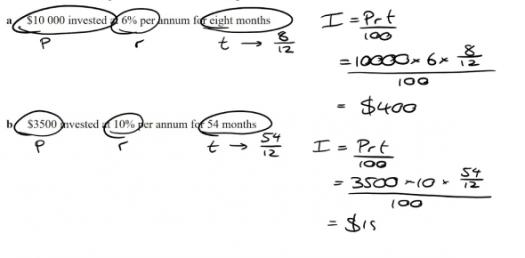# Year 12 Further Mathematics Chapter 1 & 2 Review

9 Questions | Attempts: 277SettingsSummarising numerical data: the median, range, IQR and box plots

• 1.
A sample of 21 travellers was asked the number of nights they spent away from home last year. Their responses are summarised in the following stem-and-leaf plot.0  |  1 1 2 2 3 4 5 5 6 6 7 91  |  0 2 4 4 62  |  2 2 33  |4  |  4The median number of nights away from home is:
• A.

6

• B.

6.5

• C.

7

• D.

8

• E.

9

• 2.
A sample of 21 travellers was asked the number of nights they spent away from home last year. Their responses are summarised in the following stem-and-leaf plot.0  |  1 1 2 2 3 4 5 5 6 6 7 91  |  0 2 4 4 62  |  2 2 33  |4  |  4The interquartile range (IQR) of nights away from home is:
• A.

3.5

• B.

11.5

• C.

15

• D.

22

• E.

43

• 3.
The amount of money (in dollars) spent by each of 15 students on leisure activities in the past week is as follows:10    30    30    30    35    38    45    45    47    49    50    51    52    58    100The 5 figure summary for this data is:
• A.

Minimum = 10, Q1 = 30, Median = 45, Q3 = 52, Maximum = 100

• B.

Minimum = 10, Q1 = 30, Median = 46, Q3 = 51, Maximum = 100

• C.

Minimum = 10, Q1 = 30, Median = 45, Q3 = 51.5, Maximum = 100

• D.

Minimum = 10, Q1 = 30, Median = 45, Q3 = 51, Maximum = 100

• 4.
The amount of money (in dollars) spent by each of 15 students on leisure activities in the past week is as follows:10    30    30    30    35    38    45    45    47    49    50    51    52    58    100which of the following statements is true?
• A.

50% of students spent between 30 and 51 dollars

• B.

25% of students spent less than \$35

• C.

25% of students spent more than \$50

• D.

75% of students spent less than \$50

• 5.
The amount of money (in dollars) spent by each of 15 students on leisure activities in the past week is as follows:10    30    30    30    35    38    45    45    47    49    50    51    52    58    100The data value of \$100 is an outlier.
• A.

True

• B.

False

• 6.
Two hundred people were asked about their attitude to compulsory voting (support, no opinion, do not support) and their age (in years).The variables Attitude to compulsory voting and Age are:
• A.

Both categorical variables

• B.

Both numerical variables

• C.

A categorical and a numerical variable respectively

• D.

A numerical and a categorical variable respectively

• E.

Neither numerical nor categorical variables

• 7.
Two hundred people were asked about their attitude to compulsory voting (support, no opinion, do not support) and their age (in years).The most appropriate way to graphically display the information about Age is to use a:
• A.

Dot plot

• B.

Bar chart

• C.

Segmented bar chart

• D.

Histogram

• E.

Back-to-back stem plot

• 8.
Which of the following statements is correct.
• A.

The above box plot has a symmetric distribution.

• B.

The above box plot has a negatively skewed distribution.

• C.

The above box plot has a positively skewed distribution.

• D.

The above box plot has an increasingly positive distribution.

• 9.
This distribution is approximately _________ .

## Related TopicsBack to top
×

Wait!
Here's an interesting quiz for you.